## Robert Harlander

 phone: +49-241-80-27045 fax: +49-241-80-22187 harlander(at)physik.rwth-aachen.de Büro: 28A414, Campus Melaten

Home

Brief CV

Research

Teaching

Outreach

Conferences

– Software –

Press

collaborations:

LHCHWG

LHC and Philosophy

P3H

DFG RTG

[Up: aximate]

## having fun with aximate

 The "jumping centipede": ``` \$num = 10; \$norm = 0; \$damp = 1.4; foreach \$i (1..\$num) { \$norm += 1/sqrt(\$damp**\$i); } \$xx = -120; \$nballs = 16; \$height = 80; foreach (1..\$nballs) { \$bound1 = 0; \$bound2 = 0; \$bound3 = 0; \$height += 40/\$nballs; \$xx += 15; \$i=0; while (\$bound2 < 100) { \$i++; \$bound2 += 100/\$norm/sqrt(\$damp**\$i)*sqrt(\$height/100); \$bound3 = \$bound1 + 100/\$norm/sqrt(\$damp**\$i)/2*sqrt(\$height/100); point({"coordinates" => [[\$xx,-50],[\$xx,\$height/\$damp**(\$i-1)-50]], "interval" => [\$bound1,\$bound3]}, {"coordinates" => ['\$t','(1-\$t)**2']}); point({"coordinates" => [[\$xx,\$height/\$damp**(\$i-1)-50],[\$xx,-50]], "interval" => [\$bound3,\$bound2], "staythere" => 0}, {"coordinates" => ['\$t','\$t**2']}); \$bound1 = \$bound2; } } ```An example from physics: elliptically polarized wave: ``` \$p2 = point({"type" => "empty", "coordinates" => [[50,50],[-100,-100]]}); track("c1",{%{\$p2},"color" => "Blue"}); \$total = 10; foreach \$n (0..(\$total-1)) { \$interval = [100/\$total*\$n,100], \$subtr = 1-1/\$total*\$n; \$p1 = point({"type" => "empty", "coordinates" => [[]], "interval" => \$interval}, {"coordinates" => ['50*cos(3*pi*\$t*'.\$subtr. ') + 50 - 150*(\$t*'.\$subtr.')', '70*sin(3*pi*(\$t*'.\$subtr. '))+ 50 - 150*(\$t*'.\$subtr.')']}); track("cx".\$n,{%{\$p1},"color" => "Red"}); line({"arrow" => 1, "foot" => [[]], "head" => [[]], "interval" => \$interval }, {"foot" => ['50-150*(\$t*'.\$subtr.')', '50-150*(\$t*'.\$subtr.')'], "head" => ['50*cos(3*pi*(\$t*'.\$subtr. ')) + 50 - 150*(\$t*'.\$subtr.')', '70*sin(3*pi*(\$t*'.\$subtr. '))+ 50 - 150*(\$t*'.\$subtr.')']}); } ```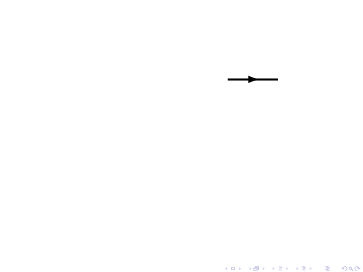Here we are randomly distributing 1000 points. However, we want to do this only in the first frame of the movie. The rest is done by the evolution. The variable \$aximate::IGLOB counts the frames, starting from 0. ``` foreach \$i (0..1000) { if (\$aximate::IGLOB==1) { \$r = 100*sqrt(rand(1)); \$phi = 2*pi*rand(1); @xpos1 = (@xpos1,\$r*cos(\$phi)); @ypos1 = (@ypos1,\$r*sin(\$phi)); @ampx = (@ampx,50*rand(1)); @ampy = (@ampy,50*rand(1)); @phase = (@phase,2*pi*rand(1)); } point({"type" => "ccirc", "color" => "Red", "bethere" => 0, "radius" => [], "coordinates" => [[]], "polar" => [[]]}, {"coordinates" => [\$xpos1[\$i].'+'.\$ampx[\$i].'*sin(2*pi*\$t+'. \$phase[\$i].')', \$ypos1[\$i].'+'.\$ampy[\$i].'*cos(2*pi*\$t+'. \$phase[\$i].')']}); } ```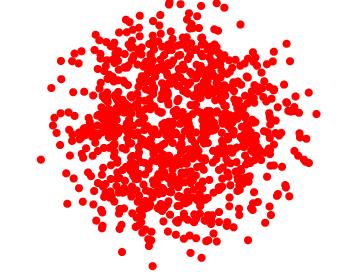## And some more, without source code...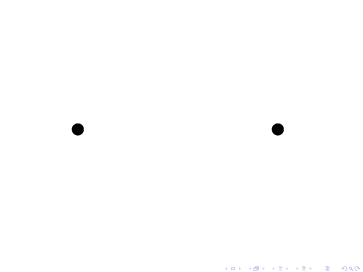stoss1 – a simple scattering reaction basic elements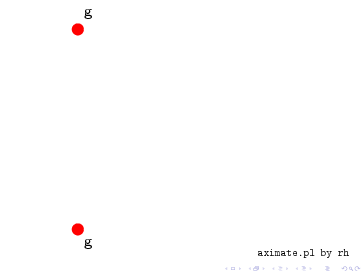ggh1 – a Feynman diagram (gluon fusion) connecting objects; attaching textwheel1 – a spinning wheel using do-loops; "circular"=1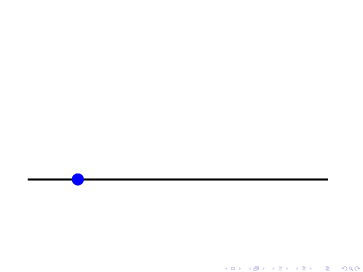wurf1 – throwing a ball changing time-evolutionfallingdisk1 – falling disk just for fun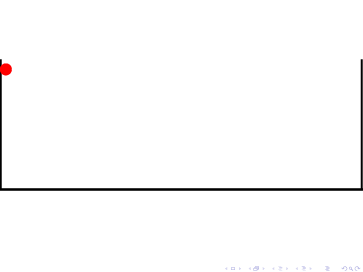jumpingball2 – jumping ball do-loop; evolve; "loop"=1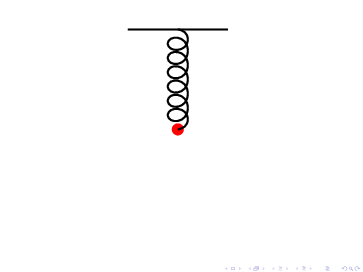federpendel1 – mass on a spring evolve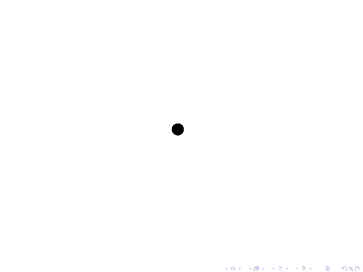spiral1 – polar coordinatesfadenpendel1 – mass on a string evolve
main
aximate:fun (Robert Harlander)